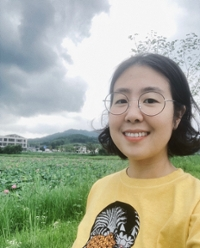# Yena Kim PH.D.

##### College of Natural and Computational Sciences - Department of Mathematics

Assistant Professor of Mathematics

EDUCATION:

• Ph.D., Mathematics, University of Florida
• M.S., Mathematics, University of Florida
• B.S., Mathematics, Washington State University

COURSES TAUGHT:

• MATH1123, Statistics
• MATH1130, Pre-calculus 1
• MATH2214, Calculus 1
• MATH2215, Calculus 2
• MATH2216, Calculus 3
• MATH3307, Differential Equations
• MATH3500, Numerical Methods
• MATH4470, Partial Differential Equations
• MATH4471, Application of Differential Equations

RESEARCH INTERESTS:

• Biomath- Epidemiological model, Immunological model, Immuno-Epidemiological model
• ODE
• PDE
• Numerical Analysis

PROFESSIONAL INTERESTS:

• Teaching Methods
• Mathematics Education

PERSONAL INTERESTS:

• Tennis
• Cooking
• Baking
• TravelingAssistant Professor
PH.D.

Send email
(808) 236-5837
500 Ala Moana Blvd. WP 4-200-R